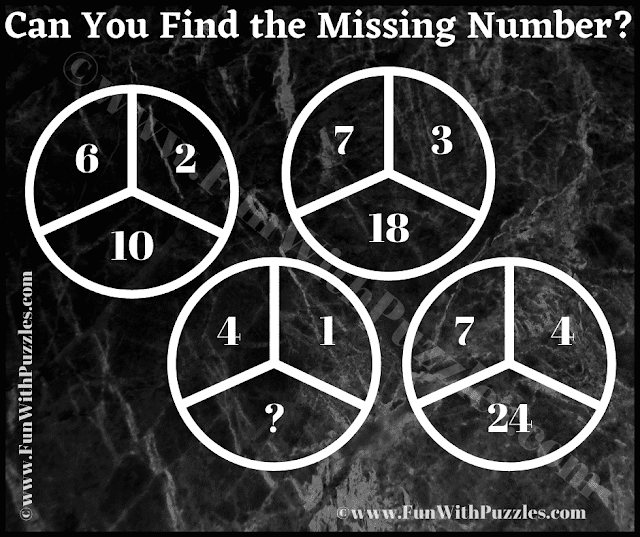This is the hard reasoning riddle that will twist your brain. In this Logic Puzzle Question, you are shown 3 numbers in the Circle. These numbers relate to each other with some logical code or mathematical formula. Your challenge in this puzzle question to break this code and find the mathematical formula which relates the number inside the circle. This is a hard logical reasoning puzzle question and will require different mathematical combinations to solve the puzzle. Can you take this challenge and Crack the Code. If you are able to solve this puzzle and find the logical reasoning used in this puzzle, please post your answer in the comments section along with your reasoning.Can you Find the Missing Number?

The answer to this "Reasoning Riddle for Adults", can be viewed by clicking on the answer button.

#### 1 comment:

Anonymous said...

C = (4x1) - 1
= 4-1
= 3